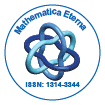# Mathematica EternaOpen Access

ISSN: 1314-3344

## Abstract

### The Explicit Solution to the Countable Systems of Linear Ordinary Differential Equations with Constant Coefficients

Mahmoud Almanassra and Iyad Suwan

In this paper, we have derived an explicit solution to an infinite countable system of linear ordinary differential equations with constant coefficients. We applied some mathematical techniques and Mathematical Induction method and have found the solution to the system under consideration when the coefficients matrix is bidiagonal.

Top